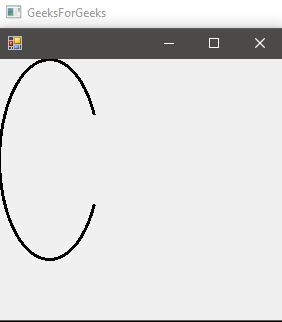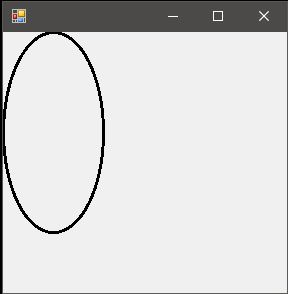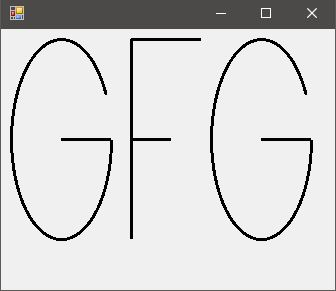# Graphics.DrawArc() Method in C# with Examples

Graphics.DrawArc Method is used to draw an arc representing a portion of an ellipse specified by a pair of coordinates, a width, and a height. There are 4 methods in the overload list of this method as follows:

• DrawArc(Pen, Rectangle, Single, Single) Method
• DrawArc(Pen, RectangleF, Single, Single) Method
• DrawArc(Pen, Int32, Int32, Int32, Int32, Int32, Int32) Method
• DrawArc(Pen, Single, Single, Single, Single, Single, Single) Method

#### DrawArc(Pen, Rectangle, Single, Single)

This method is used to draw an arc representing a portion of an ellipse specified by a Rectangle structure.

Syntax: public void DrawArc (System.Drawing.Pen pen, System.Drawing.Rectangle rect, float startAngle, float sweepAngle)

Parameters:
pen: Determines the color, width, and style of the arc.
rect: Determines the smallest rectangle in which the ellipse just perfectly fits into.
startAngle: Angle in degrees measured clockwise from the x-axis to the starting point of the arc.
sweepAngle: Angle in degrees measured clockwise from the startAngle parameter to ending point of the arc.

Exception: This method will give ArgumentNullException if the pen is null.

Example:

 `// C# program to illustrate the  ` `// DrawArc(Pen, Rectangle, Single, ` `// Single) Method ` `using` `System; ` `using` `System.Drawing; ` `using` `System.Drawing.Printing; ` `using` `System.Windows.Forms; ` ` `  `namespace` `GFG { ` ` `  `class` `PrintableForm : Form { ` ` `  `    ``// Main Method ` `    ``public` `static` `void` `Main() ` `    ``{ ` `        ``Application.Run(``new` `PrintableForm()); ` `    ``} ` `    ``public` `PrintableForm() ` `    ``{ ` `        ``ResizeRedraw = ``true``; ` `    ``} ` ` `  `    ``protected` `override` `void` `OnPaint(PaintEventArgs e) ` `    ``{ ` `        ``// Create pen. ` `        ``Pen blackPen = ``new` `Pen(Color.Black, 3); ` ` `  `        ``// Reactangle with specifies x1, ` `        ``// y1, x2, y2 respectively ` `        ``Rectangle rect = ``new` `Rectangle(0, 0, 100, 200); ` ` `  `        ``// Create start and sweep angles on ellipse. ` `        ``float` `startAngle = 45.0F; ` `        ``float` `sweepAngle = 270.0F; ` ` `  `        ``// Draw arc to screen. ` `        ``e.Graphics.DrawArc(blackPen, rect,  ` `                  ``startAngle, sweepAngle); ` `    ``} ` `} ` `} `

Output:#### DrawArc(Pen, RectangleF, Single, Single)

This method is used to draw an arc representing a portion of an ellipse specified by a RectangleF structure.

Syntax: public void DrawArc (System.Drawing.Pen pen, System.Drawing.RectangleF rect, float startAngle, float sweepAngle);

Parameters:
pen: Determines the color, width, and style of the arc.
rect: Determines the smallest rectangle in which the ellipse just perfectly fits into.
startAngle: Angle in degrees measured clockwise from the x-axis to the starting point of the arc.sweepAngle: Angle in degrees measured clockwise from the startAngle parameter to ending point of the arc.

Exception: This method will give ArgumentNullException if the pen is null.

Example 1:

 `// C# program to illustrate the  ` `// DrawArc(Pen, RectangleF,  ` `// Single, Single) Method ` `using` `System; ` `using` `System.Drawing; ` `using` `System.Drawing.Printing; ` `using` `System.Windows.Forms; ` ` `  `namespace` `GFG { ` ` `  `class` `PrintableForm : Form { ` ` `  `    ``// Main Method ` `    ``public` `static` `void` `Main() ` `    ``{ ` ` `  `        ``Application.Run(``new` `PrintableForm()); ` `    ``} ` ` `  `    ``public` `PrintableForm() ` `    ``{ ` `        ``ResizeRedraw = ``true``; ` `    ``} ` ` `  `    ``protected` `override` `void` `OnPaint(PaintEventArgs e) ` `    ``{ ` `        ``// Create pen ` `        ``Pen blackPen = ``new` `Pen(Color.Black, 3); ` ` `  `        ``// Reactangle with specifies x1, ` `        ``// y1, x2, y2 respectively ` `        ``RectangleF rect = ``new` `RectangleF(0.0F,  ` `                        ``0.0F, 100.0F, 200.0F); ` ` `  `        ``// Create start and sweep ` `        ``// angles on an ellipse. ` `        ``float` `startAngle = 45.0F; ` `        ``float` `sweepAngle = 270.0F; ` ` `  `        ``// Draw arc to screen. ` `        ``e.Graphics.DrawArc(blackPen, rect, ` `                  ``startAngle, sweepAngle); ` `    ``} ` `} ` `} `

Output:#### DrawArc(Pen, Single, Single, Single, Single, Single, Single)

This method is used to draw an arc representing a portion of an ellipse specified by a pair of coordinates, a width, and a height.

Syntax: public void DrawArc (System.Drawing.Pen pen, float x, float y, float width, float height, float startAngle, float sweepAngle);

Parameters:
pen: Pen determines the color, width, and style of the line.
x: The abscissa of the upper-left corner of the rectangle that defines the ellipse.
y: The ordinate of the upper-left corner of the rectangle that defines the ellipse.
width: Width of the rectangle that defines the ellipse or more specifically we can say the diameter of the ellipse in abscissa.
height: Height of the rectangle that defines the ellipse or more specifically we can say the diameter of the ellipse in ordinate.
startAngle: Angle in degrees measured clockwise from the x-axis to the starting point of the arc.
sweepAngle: Angle in degrees measured clockwise from the startAngle parameter to the ending point of the arc.

Exception: This method will give ArgumentNullException if the pen is null.

Example 1:

 `// C# program to draw a circle ` `using` `System; ` `using` `System.Drawing; ` `using` `System.Drawing.Printing; ` `using` `System.Windows.Forms; ` ` `  `namespace` `GFG { ` ` `  `class` `PrintableForm : Form { ` ` `  `    ``// Main Method ` `    ``public` `static` `void` `Main() ` `    ``{ ` `        ``Application.Run(``new` `PrintableForm()); ` `    ``} ` ` `  `    ``public` `PrintableForm() ` `    ``{ ` `        ``ResizeRedraw = ``true``; ` `    ``} ` ` `  `    ``protected` `override` `void` `OnPaint(PaintEventArgs e) ` `    ``{ ` `        ``// Create pen. ` `        ``Pen blackPen = ``new` `Pen(Color.Black, 3); ` ` `  `        ``// Create coordinates of the rectangle ` `        ``// to the bound ellipse. ` `        ``int` `x = 0; ` `        ``int` `y = 0; ` `        ``int` `width = 100; ` `        ``int` `height = 200; ` ` `  `        ``// Create start and sweep  ` `        ``// angles on ellipse. ` `        ``int` `startAngle = 0; ` `        ``int` `sweepAngle = 360; ` ` `  `        ``// Draw arc to screen. ` `        ``e.Graphics.DrawArc(blackPen, x, y, width, ` `                 ``height, startAngle, sweepAngle); ` `    ``} ` `} ` `} `

Output:Example 2:

 `// C# program to draw GFG ` `using` `System; ` `using` `System.Drawing; ` `using` `System.Drawing.Printing; ` `using` `System.Windows.Forms; ` ` `  `namespace` `GFG { ` ` `  `class` `PrintableForm : Form { ` ` `  `    ``// Main Method ` `    ``public` `static` `void` `Main() ` `    ``{ ` `        ``Application.Run(``new` `PrintableForm()); ` `    ``} ` ` `  `    ``public` `PrintableForm() ` `    ``{ ` `        ``ResizeRedraw = ``true``; ` `    ``} ` ` `  `    ``protected` `override` `void` `OnPaint(PaintEventArgs e) ` `    ``{ ` `        ``// Create pen. ` `        ``Pen blackPen = ``new` `Pen(Color.Black, 3); ` ` `  `        ``// Creates letter G ` `        ``e.Graphics.DrawArc(blackPen, 10, ` `                  ``10, 100, 200, 0, 315); ` ` `  `        ``e.Graphics.DrawLine(blackPen, ` `                  ``60, 110, 110, 110); ` ` `  `        ``// Creates letter F ` `        ``e.Graphics.DrawLine(blackPen, ` `                  ``130, 10, 130, 210); ` ` `  `        ``e.Graphics.DrawLine(blackPen, ` `                   ``130, 10, 200, 10); ` ` `  `        ``e.Graphics.DrawLine(blackPen,  ` `                 ``130, 110, 170, 110); ` ` `  `        ``// Creates the next letter G ` `        ``e.Graphics.DrawArc(blackPen, ` `         ``210, 10, 100, 200, 0, 315); ` ` `  `        ``e.Graphics.DrawLine(blackPen, ` `                 ``260, 110, 310, 110); ` `    ``} ` `} ` `} `

Output:#### DrawArc(Pen, Int32, Int32, Int32, Int32, Int32, Int32)

This method is used to draw an arc representing a portion of an ellipse specified by a pair of coordinates, a width, and a height.

Syntax: public void DrawArc (System.Drawing.Pen pen, int x, int y, int width, int height, int startAngle, int sweepAngle);

Parameters:
pen: Pen determines the color, width, and style of the line.
x: The abscissa of the upper-left corner of the rectangle that defines the ellipse.
y: The ordinate of the upper-left corner of the rectangle that defines the ellipse.
width: Width of the rectangle that defines the ellipse or more specifically we can say the diameter of the ellipse in abscissa.
height: Height of the rectangle that defines the ellipse or more specifically we can say the diameter of the ellipse inordinate.
startAngle: Angle in degrees measured clockwise from the x-axis to the starting point of the arc.
sweepAngle: Angle in degrees measured clockwise from the startAngle parameter to ending point of the arc.

Exception: This method will give ArgumentNullException if the pen is null.

Example:

 `// C# program to draw a circle ` `using` `System; ` `using` `System.Drawing; ` `using` `System.Drawing.Printing; ` `using` `System.Windows.Forms; ` ` `  `namespace` `GFG { ` ` `  `class` `PrintableForm : Form { ` ` `  `    ``// Main Method ` `    ``public` `static` `void` `Main() ` `    ``{ ` `        ``Application.Run(``new` `PrintableForm()); ` `    ``} ` `    ``public` `PrintableForm() ` `    ``{ ` `        ``ResizeRedraw = ``true``; ` `    ``} ` `    ``protected` `override` `void` `OnPaint(PaintEventArgs e) ` `    ``{ ` `        ``// Create pen. ` `        ``Pen blackPen = ``new` `Pen(Color.Black, 3); ` ` `  `        ``// Create coordinates of the rectangle ` `        ``// to the bound ellipse. ` `        ``float` `x = 0.0F; ` `        ``float` `y = 0.0F; ` `        ``float` `width = 100.0F; ` `        ``float` `height = 200.0F; ` ` `  `        ``// Create start and sweep ` `        ``// angles on the ellipse. ` `        ``float` `startAngle = 0.0F; ` `        ``float` `sweepAngle = 360.0F; ` ` `  `        ``// Draw arc to screen. ` `        ``e.Graphics.DrawArc(blackPen, x, y, width, ` `                 ``height, startAngle, sweepAngle); ` `    ``} ` `} ` `} `

Output:Reference:

My Personal Notes arrow_drop_upCheck out this Author's contributed articles.

If you like GeeksforGeeks and would like to contribute, you can also write an article using contribute.geeksforgeeks.org or mail your article to contribute@geeksforgeeks.org. See your article appearing on the GeeksforGeeks main page and help other Geeks.

Please Improve this article if you find anything incorrect by clicking on the "Improve Article" button below.

Article Tags :

Be the First to upvote.

Please write to us at contribute@geeksforgeeks.org to report any issue with the above content.